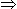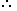# Tips and Tricks on Simplification related problems in Aptitude1. ‘BODMAS’ Rule:This rule depicts the correct sequence in which the operations are to be executed, so as to find out the value of given expression.Here B – Bracket,
O – of,
D – Division,
M – Multiplication,
S – Subtraction

Thus, in simplifying an expression, first of all the brackets must be removed, strictly in the order (), {} and ||.

After removing the brackets, we must use the following operations strictly in the order:

(i) of (ii) Division (iii) Multiplication (iv) Addition (v) Subtraction.

2. Modulus of a Real Number:Modulus of a real number a is defined as
 |a| =a, if a > 0 –a, if a < 0

Thus, |5| = 5 and |-5| = -(-5) = 5.

3. Virnaculum (or Bar):When an expression contains Virnaculum, before applying the ‘BODMAS’ rule, we simplify the expression under the Virnaculum
A man has Rs. 480 in the denominations of one-rupee notes, five-rupee notes and ten-rupee notes. The number of notes of each denomination is equal. What is the total number of notes that he has ?
 A. 45 B. 60 C. 75 D. 90

Explanation:

Let number of notes of each denomination be x.

Then x + 5x + 10x = 48016x = 480x = 30.

Hence, total number of notes = 3x = 90.

2. There are two examinations rooms A and B. If 10 students are sent from A to B, then the number of students in each room is the same. If 20 candidates are sent from B to A, then the number of students in A is double the number of students in B. The number of students in room A is:
 A. 20 B. 80 C. 100 D. 200

Explanation:

Let the number of students in rooms A and B be x and y respectively.

Then, x – 10 = y + 10x – y = 20 …. (i)

and x + 20 = 2(y – 20)x – 2y = -60 …. (ii)

Solving (i) and (ii) we get: x = 100 , y = 80.The required answer A = 100.

#### The price of 10 chairs is equal to that of 4 tables. The price of 15 chairs and 2 tables together is Rs. 4000. The total price of 12 chairs and 3 tables is:

Correct! Wrong!

#### The price of 2 sarees and 4 shirts is Rs. 1600. With the same money one can buy 1 saree and 6 shirts. If one wants to buy 12 shirts, how much shall he have to pay ?

Correct! Wrong!

#### A sum of Rs. 1360 has been divided among A, B and C such that A gets of what B gets and B gets of what C gets. B's share is:A sum of Rs. 1360 has been divided among A, B and C such that A gets of what B gets and B gets of what C gets. B's share is:A sum of Rs. 1360 has been divided among A, B and C such that A gets of what B gets and B gets of what C gets. B's share is:A sum of Rs. 1360 has been divided among A, B and C such that A gets of what B gets and B gets of what C gets. B's share is:V

Correct! Wrong!

#### One-third of Rahul's savings in National Savings Certificate is equal to one-half of his savings in Public Provident Fund. If he has Rs. 1,50,000 as total savings, how much has he saved in Public Provident Fund ?

Correct! Wrong!

#### A fires 5 shots to B's 3 but A kills only once in 3 shots while B kills once in 2 shots. When B has missed 27 times, A has killed:

Correct! Wrong!

QUIZ ON SIMPLIFICATION RELATED PROBLEMS
EXCELLENT!!!